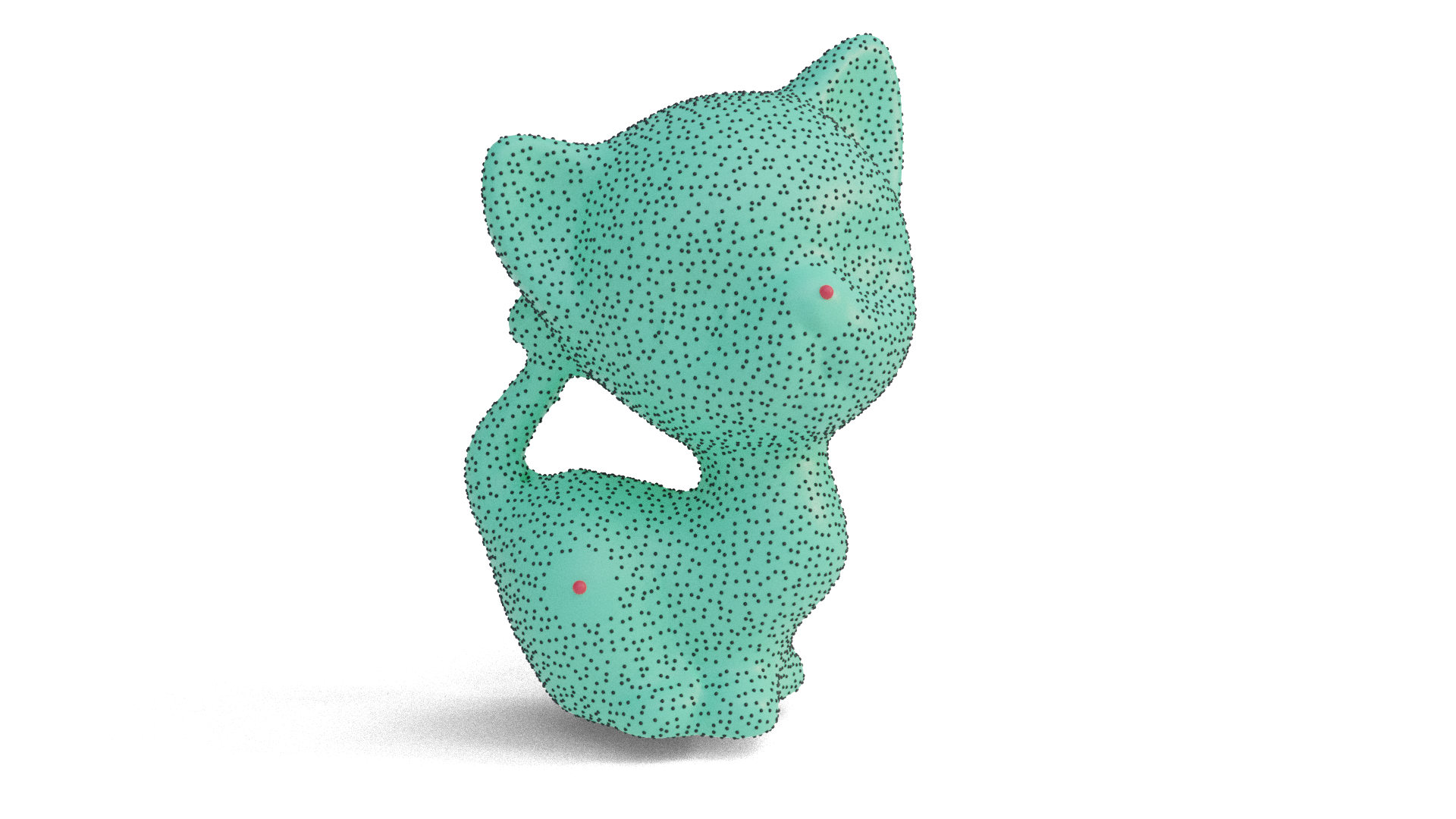# Surface Sampling

## Poisson disk sampling

The PoissonDiskSampler class contains a function that Poisson disk-samples a surface mesh so that each pair of sampled points are at a minimum distance r away from each other. This ensures that sampled points are distributed evenly in 3D space. Technically, Poisson disk sampling on surfaces should ensure that points are at least a certain geodesic distance away from each other. But for the purposes of visualization, it can be better to use extrinsic distance of the embedded surface instead, since this will ensure that the sampling looks even – two points could have a large geodesic distance between them but be very near each other in 3D space, which might lead to a visually crowded image.

Currently the algorithm only works on manifold meshes.

#include "geometrycentral/surface/poisson_disk_sampler.h"

The algorithm has a few parameters that roughly correspond to the algorithm of Bridson’s 2007 Fast Poisson Disk Sampling in Arbitrary Dimensions.

Additionally, you can specify points around samples should be avoided (shown in red below.) By default, samples will avoid these points with the same radius r used in the rest of the algorithm. You can optionally specify a “radius of avoidance” for these points, where the radius of avoidance is given in multiples of r.

The radius of avoidance can be further be specified to be a radius in 3D space, or a radius in terms of distance along the surface. The former will produce a radius of avoidance that will appear perfectly round and is likely more visually pleasing, but for very large radii may occlude samples from opposite sides of the mesh. The latter will restrict the radius of avoidance to only be along the surface, but such a metric ball will not appear perfectly round, especially in areas with very large and sudden changes in curvature.PoissonDiskSampler::PoissonDiskSampler(ManifoldSurfaceMesh& mesh, VertexPositionGeometry& geometry)

Create a new solver to Poisson disk-sample on the given mesh with the given geometry.

• mesh specifies the mesh. It must be manifold, since the sampling depends on the traceGeodesic function.
• geometry specifies the VertexPositionGeometry needed to describe the positions of vertices in 3D space.

The mesh and geometry cannot be changed after construction.

std::vector<SurfacePoint> PoissonDiskSampler::sample(double rCoef = 1.0, int kCandidates = 30, std::vector<SurfacePoint> pointsToAvoid = std::vector<SurfacePoint>(), int rAvoidance = 1, bool use3DAvoidanceRadius = true);

Poisson disk-sample the surface mesh.

• rCoef: corresponds to the minimum distance between samples, expressed as a multiple of the mean edge length. The actual minimum distance is computed as r = rCoef * meanEdgeLength
• kCandidates: the number of candidate points chosen from the (r,2r)-annulus around each sample.
• pointsToAvoid: SurfacePoints which samples should avoid.
• rAvoidance: the radius of avoidance around each point to avoid, expressed as a multiple of r.
• use3DAvoidanceRadius: If true, the radius of avoidance will specify a solid ball in 3D space around which samples are avoided. Otherwise, samples are avoided within a ball on the surface.

### Example

#include "geometrycentral/surface/manifold_surface_mesh.h"
#include "geometrycentral/surface/vertex_position_geometry.h"
#include "geometrycentral/surface/meshio.h"
#include "geometrycentral/surface/surface_point.h"
#include "geometrycentral/surface/poisson_disk_sampler.h"